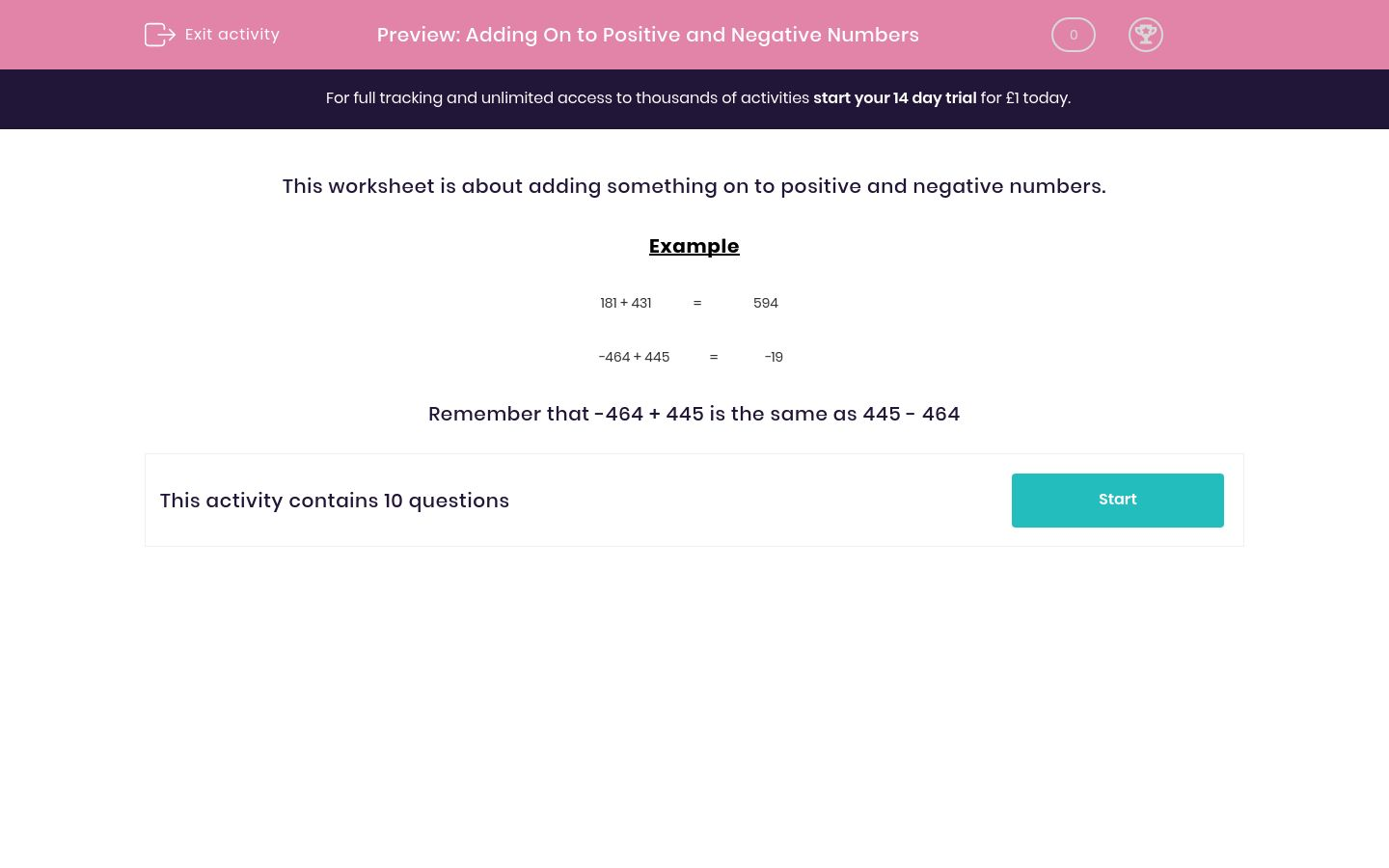# Adding On to Positive and Negative Numbers

In this worksheet, students add a number on to positive and negative numbers.Key stage:  KS 2

Curriculum topic:   Maths and Numerical Reasoning

Curriculum subtopic:   Mixed Problems

Difficulty level:### QUESTION 1 of 10

This worksheet is about adding something on to positive and negative numbers.

Example

181 + 431 =
 594

−464 + 445 =
 −19

Remember that -464 + 445 is the same as 445 - 464

---- OR ----